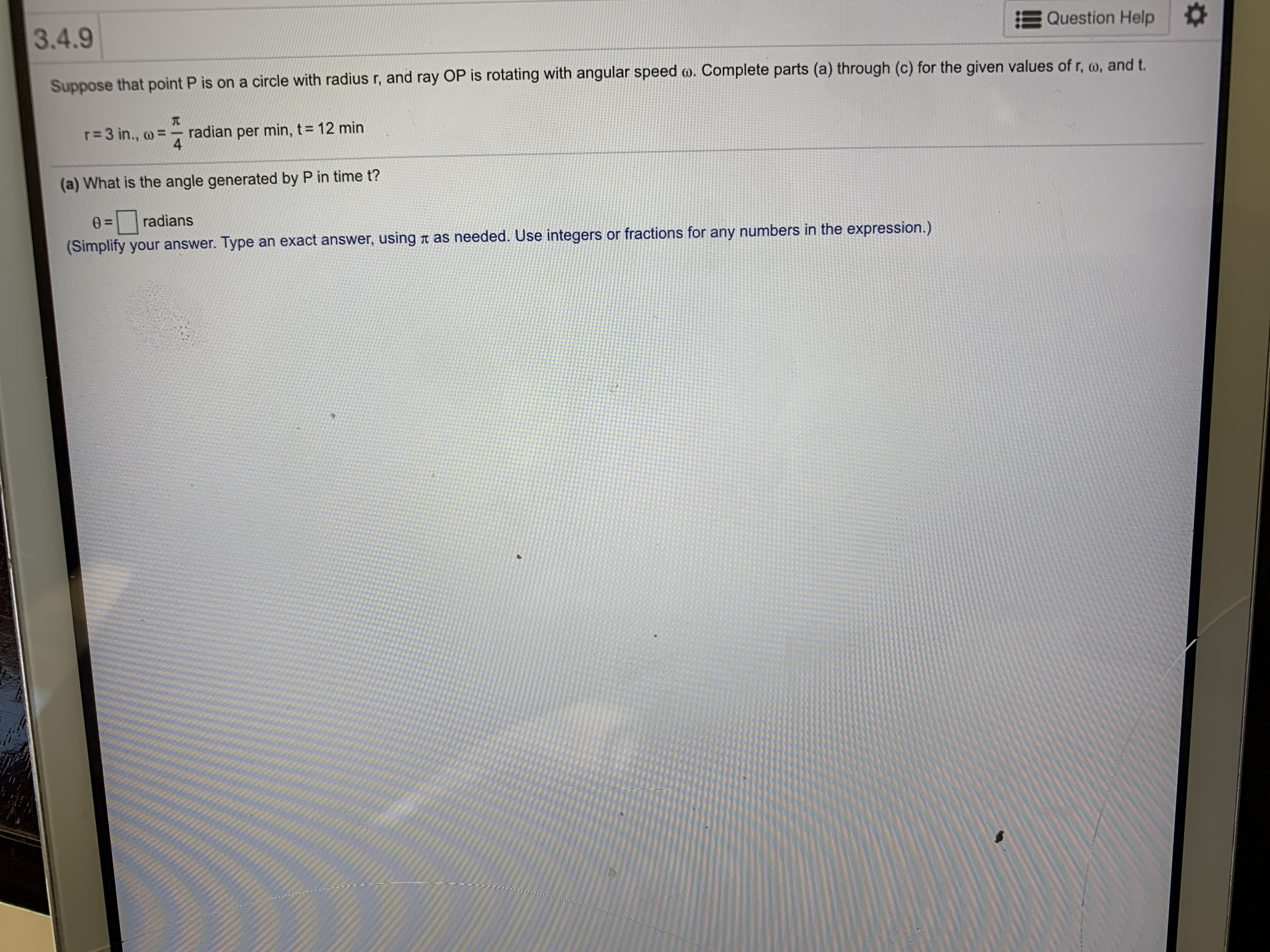# 3.4.9Question HelpSuppose that point P is on a circle with radius r, and ray OP is rotating with angular speed o. Complete parts (a) through (c) for the given values of r, o, and t.r=3 in., oradian4min, t 12 minper(a) What is the angle generated by P in time t?0 =radians(Simplify your answer. Type an exact answer, using t as needed. Use integers or fractions for any numbers in the expression.)

Question
6 viewshelp_outlineImage Transcriptionclose3.4.9 Question Help Suppose that point P is on a circle with radius r, and ray OP is rotating with angular speed o. Complete parts (a) through (c) for the given values of r, o, and t. r=3 in., o radian 4 min, t 12 min per (a) What is the angle generated by P in time t? 0 = radians (Simplify your answer. Type an exact answer, using t as needed. Use integers or fractions for any numbers in the expression.) fullscreen
check_circle

Given angular speed is ꞷ=  radians per min

Given time is t=12 mi...

### Want to see the full answer?

See Solution

#### Want to see this answer and more?

Solutions are written by subject experts who are available 24/7. Questions are typically answered within 1 hour.*

See Solution
*Response times may vary by subject and question.
Tagged in

### Trigonometry